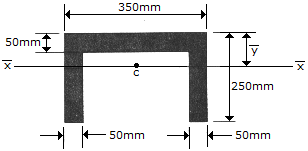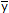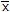# Engineering Mechanics - Center of Gravity and Centroid - Discussion

### Discussion :: Center of Gravity and Centroid - General Questions (Q.No.5)

5.Determine the distanceto the centroidal axisof the beam's cross-sectional area.

 [A].= 112.3 mm [B].= 125.0 mm [C].= 100.0 mm [D].= 91.7 mm

Explanation:

No answer description available for this question.

 Ahamed said: (Oct 8, 2011) Since the given figure is symmetric about y-axis So therefore x' = 0 and y' = (A1y1 + A2y2 + A3y3) / ( A1 + A2 +A3) A1 = 350 X 50 = 17500 mm A2 = 200 X 50 = 10000 mm A3 = 200 X 50 = 10000 mm y1 = 25 y2 = 50+100 = 150 y3 = 50+100 = 150 y' = (17500x25 + 10000x150 + 10000x150) / (17500 + 10000 + 10000) y' = 91.6666666 mm By rounding off we get, y' = 91.7 mm.

 Danica said: (Oct 10, 2015) Why does y1 didn't consider the 350?

 Sai Lakshmi said: (Dec 4, 2015) Why didn't you do division for y2?

 Ali said: (Aug 25, 2016) Please solve the question clearly by parts.

 Ali J. said: (May 25, 2017) Good, Thanks @Ahamed.

 Upasana said: (Apr 2, 2018) Please, solve the question clearly.